• java整数拆分
2022-04-30 10:03:45

给定一个正整数 n ，将其拆分为 k正整数 的和（ k >= 2 ），并使这些整数的乘积最大化。

返回 你可以获得的最大乘积

提示:

• 2 <= n <= 58
• ### 1、数学归纳

class Solution {
public int integerBreak(int n) {
if(n <= 3) return n - 1;
int a = n / 3, b = n % 3;
if(b == 0) return (int)Math.pow(3, a);
if(b == 1) return (int)Math.pow(3, a - 1) * 4;
return (int)Math.pow(3, a) * 2;
}
}

2、动态规划

class Solution {
public int integerBreak(int n) {
int[] dp = new int[n+1];
Arrays.fill(dp,-1);
dp = 1;

for(int i = 3;i < n+1;i++){
int curMax = 0;
for(int j = 1;j < i;j++){
curMax = Math.max(curMax,Math.max(j*(i-j),dp[i-j]*j));
//curMax = Math.max(curMax,j*(i-j));
}
dp[i] = curMax;
}
return dp[n];
}
}leetcode 算法 数据结构
更多相关内容
• class Solution { public int integerBreak(int n) { int []dp=new int [n+1]; dp=1; for(int i=3;i<=n;i++){ for(int j=1;... dp[i]=Math.max(dp[i],Math.max(j*dp[i-j],j*(i-j)));... ...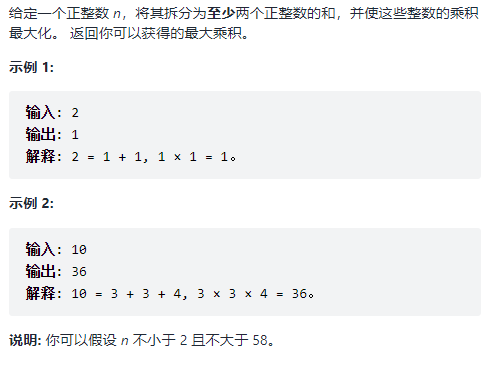class Solution {
public int integerBreak(int n) {
int []dp=new int [n+1];
dp=1;
for(int i=3;i<=n;i++){
for(int j=1;j<i-1;j++){
dp[i]=Math.max(dp[i],Math.max(j*dp[i-j],j*(i-j)));
}
}
return dp[n];
}
}

展开全文java 动态规划
• 给定一个正整数 n，将其拆分为至少两个正整数的和，并使这些整数的乘积最大化。 返回你可以获得的最大乘积。 二、动态规划分析 当前整数的最大值，是由拆分得到的正整数决定的，所以当前状态是由上一个状态推导而来...

## 一、题目

给定一个正整数 n，将其拆分为至少两个正整数的和，并使这些整数的乘积最大化。 返回你可以获得的最大乘积。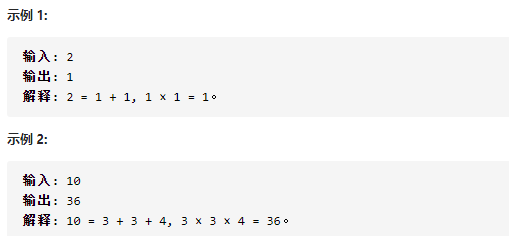## 二、动态规划分析

当前整数的最大值，是由拆分得到的正整数决定的，所以当前状态是由上一个状态推导而来，选用动态规划解决

（1）确定数组及下标含义

dp[i]：表示分拆数字 i ，可以得到的最大乘积 dp[i]

（2）确定递推公式

获得 dp[i]要么直接是(i-j)*j
要么：j*dp[i-j]
j 从1开始遍历到 i-1，所以对于 i 来说所有的情况都会遍历到在 (1,i-1)之间选择最大的 dp[i]
dp[i]=Ma

展开全文动态规划 java 算法 数据结构 leetcode
• ## Java正整数拆分算法

千次阅读 2021-02-12 11:41:14
整数拆分一、概念所谓整数拆分，是指把一个正整数拆分成若干正整数的和。拆分数即对应不同的拆分法的个数。如：正整数4的拆分数4=44=3+14=2+24=2+1+14=1+1+1+1二、模型正整数n的拆分，相当于把n个相同的球放进n...

整数的拆分

一、概念

所谓整数的拆分，是指把一个正整数拆分成若干正整数的和。

拆分数即对应不同的拆分法的个数。

如：正整数4的拆分数

4=4

4=3+1

4=2+2

4=2+1+1

4=1+1+1+1

二、模型

正整数n的拆分，相当于把n个相同的球放进n个相同的盒子里，盒子中可以有一个以上的球，也可以空着。还以正整数4为例，球的放法如下：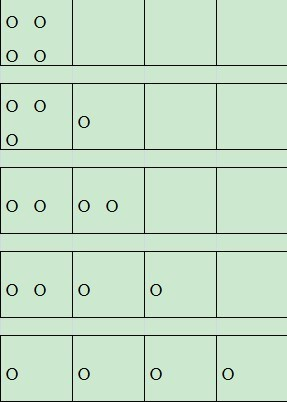三、算法：

定义一个方法Q(sum, max)，表示整数sum所有加数都不超过max的拆分数

sum的拆分数就表示为Q(sum, sum)

递归关系如下：

1、Q(sum, sum) = 1 + Q(sum, sum)

2、Q(sum, max) = Q(sum-max, max) + Q(sum, max-1)

3、Q(sum, 1) = 1或者Q(1,max) = 1， 停止。

四、java代码实现

package temporary;

public class SplitInteger {

/**

* 正整数加法不同的分解法

* @param sum：和

* @param max：最大值

* @param data：记录不同的加法形式

* @param index：加法分解数的最大个数

* @return 分解个数

*/

public static int splitInteger(int sum, int max, int[] data, int index) {

if (max > sum) max = sum;

if (sum < 1 || max < 1) return 0;

if (sum == 1 || max == 1) {

if (sum == 1) {

data[index] = sum;

print(data, index+1);

} else {

for (int i = 0; i < sum; i++) {

data[index++] = max;

}

print(data, index);

}

return 1;

}

if (sum == max) {

data[index] = max;

print(data, index+1);

return 1 + splitInteger(sum, max-1, data, index);

} else if (sum > max) {

data[index] = max;

//一定注意这里的先后顺序

return splitInteger(sum-max, max, data, index+1) + splitInteger(sum, max-1, data, index);

} else {

//sum < max

return splitInteger(sum, sum, data, index);

}

}

//打印数组

public static void print(int[] data, int index) {

for (int i = 0; i < index -1; i++) {

System.out.print(data[i] + "+");

}

System.out.println(data[index-1]);

}

/**

* 正整数加法不同分解的个数:最大值为max，和为sum的加法个数

* 递归形式： f(sum, max) = f(sum-max, max) + f(sum, max-1);

* @param sum

* @param max

* @return

*/

public static int count(int sum, int max) {

if (sum < 1 || max < 1) return 0;

else if (sum == 1 || max == 1){

return 1;

} else if (sum < max) {

return count(sum,sum);

} else if (sum == max) {

return 1+count(sum, sum-1);

} else {

return count(sum, max-1)+count(sum-max,max);

}

}

public static void main(String[] args) {

int n = 4;

int[] data = new int[n];

System.out.println("正整数\'" + n + "\'可以分解为如下不同的加法形式：");

System.out.println("正整数\'" + n + " \'加法分解个数为：\t" + splitInteger(n,n,data,0));

n = 100;

System.out.println("正整数\'" + n + "\'加法分解个数为(包含本身)：\t" + count(n,n));

System.out.println("正整数\'" + n + "\'加法分解个数为(不包含本身)：\t" + count(n,n-1));

}

}

//output~

正整数'4'可以分解为如下不同的加法形式：

4

3+1

2+2

2+1+1

1+1+1+1

正整数'4 '加法分解个数为：5

正整数'100'加法分解个数为(包含本身)：190569292

正整数'100'加法分解个数为(不包含本身)：190569291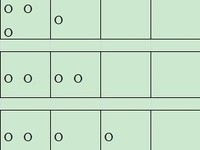大小: 16.7 KB

分享到：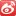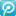2012-10-19 13:49

浏览 7940

评论

展开全文• 题目描述：给定一个一位以上的整数，要求在其中插入乘号，并得出乘积最大的一组 举例：若输入1234，则计算： 1234 1234 123*4 要求：输入必须是一位以上的正整数，重复输入三次后弹出 参考代码： 个人实现： ...java 开发语言 算法
• 1.将正整数n无序拆分成最大数为m的拆分方案个数，要求所有拆分方案不重复。样例：n = 5, m = 5,对应的拆分方案如下：5 = 55 = 4 + 15 = 3 + 25 = 3 + 1 + 15 = 2 + 2 + 15 = 2 + 1 + 1 + 15 = 1 + 1 + 1 + 1 + 1分析...
• 题目描述给定一个正整数，我们可以定义出下面的公式:N=a+a+a+…+a[m];a[i]>0,1<=m<=N;对于一个正整数，求解满足上面公式的所有算式组合，如，对于整数4 :4= 4;4 = 3 + 1;4 = 2 + 2;4 = 2 + 1 + 1;...java整数拆分算法
• 整数拆分问题， 求最有值，具有最优子结构，具有重叠自问题 --->符合动态规划模型 通过自底向下的方式，把计算过的自问题结果保存起来，减少运算次数。 /** * 整数划分问题 * 动态规划， * 要分析清楚， ...整数拆分 动态规划 Java
• 根据罗列的最大值结果，从n=5开始，拆分的结果都有数字3。 之后的数字，例如11，可以先拆出来1个3，然后再看余下的8如何拆分。 问题：为何只能取到58？ 解决：int最大值为2 的 31 次方 - 1 =2147483648 - 1=...Java
• ①所求分数小于1，且分子分母均为正整数 ②将其拆分成两个自然数的倒数和 ③在所有的可能解中，筛出两自然数不相同的情况并输出 例 ① ② 在括号中填入不同的自然数，使等式成立 思维和解法 ① 参考...java
• 输入n，和k，问将n用1到k这k个数字进行拆分，有多少种拆分方法。例如：n=5,k=3 则有n=3+2, n=3+1+1, n=2+1+1+1, n=2+2+1, n=1+1+1+1+1这5种拆分方法。这个题目是个比较明显的动态规划，如果想不到是背包问题，也可以...整数拆分算法java
• 整数拆分求最大乘积问题 问题描述：给定一个正整数 n，将其分解为至少两个正整数的总和并最大化这些整数的乘积。返回您可以得到的最大结果。 例如，给定 n = 2，返回 1 (2 = 1 + 1)； 给定 n = 10，返回 36 (10 =...算法
• 给定一个正整数 n，将其拆分为至少两个正整数的和，并使这些整数的乘积最大化。 返回你可以获得的最大乘积。 一、示意图 二、解题思路 //如果是动态规划的话 那只能是dp[n]=max(dp[n-1]*1,dp[n-2]*2)..这样子吧 ...动态规划 java 算法 leetcode
• 很明显可以用动态规划，n=2时的值是知道的（2=1+1,1×1=1）。），并使这些整数的乘积最大化。你可以获得的最大乘积。java leetcode python 算法
• @Test public void test10() { int numb = 1234; int g = numb % 10; // 个位 int s = (numb / 10) % 10;// 十位 int b = (numb / 100) % 10; // 百位 int q = (numb / 1000) % 10;... System.out.p...JavaSE
• ## 整数拆分--java/C++

千次阅读 2018-10-24 14:31:58
一个整数总可以拆分为2的幂的和，例如： 7=1+2+4 7=1+2+2+2 7=1+1+1+4 7=1+1+1+2+2 7=1+1+1+1+1+2 7=1+1+1+1+1+1+1 总共有六种不同的拆分方式。 再比如：4可以拆分成：4 = 4，4 = 1 + 1 + 1 + 1，4 = 2 + 2，4=1+1+2...计算机考研机试 整数拆分 java
• 给定一个正整数 n，将其拆分为至少两个正整数的和，并使这些整数的乘积最大化。 返回你可以获得的最大乘积。 说明: 你可以假设 n 不小于 2 且不大于 58。 样例描述 示例 1: 输入: 2 输出: 1 解释: 2 = 1 + 1, 1 × ...动态规划
• 343. 整数拆分 - 力扣（LeetCode） (leetcode-cn.com) 给定一个正整数 n ，将其拆分为 k 个 正整数 的和（ k >= 2 ），并使这些整数的乘积最大化。 返回 你可以获得的最大乘积 。 示例 1: 输入: n = 2 输出:...算法 java
• 1.题目 2.解法 ①一维数组+动态规划 class Solution { public int integerBreak(int n) { if(n == 2) return 1; if(n == 3) return 2; int[] dp = new int[n + 1]; for(int i = 2;... for(int j =动态规划 leetcode java 算法 数据结构
• 我需要编写一个方法，该方法以单个链接的整数列表和一个称为拆分值的特殊值开头 . 列表的元素没有特定的顺序 . 该方法将节点划分为两个链接列表：一个包含所有包含小于拆分值的元素的节点，另一个包含所有其他节点 ....
• Edit: Taylor L correctly remarks that though >> works in this case, it may yield incorrect results if you generalize this code to four bytes (since in Java an int is 32 bits). In that case, it's ...
• 从欧拉到拉马努金，带你从整数拆分问题了解动态规划常用的优化策略：斜率优化。算法 java 动态规划
•算法 整数拆分
• 343. 整数拆分 给定一个正整数 n ，将其拆分为 k 个 正整数 的和（ k >= 2 ），并使这些整数的乘积最大化。 返回 你可以获得的最大乘积 。 示例 1: 输入: n = 2 输出: 1 解释: 2 = 1 + 1, 1 × 1 = 1。 示例 2: ...leetcode
• import java.io.File;import java.io.FileWriter;import java.io.IOException;import java.util.*;public class aa {public static int a=0 ;public static int Devide(int input, int base, int []pData, int index...整数拆分算法java
• import java.util.ArrayList; import java.util.List; import java.util.Random; public class TestCat { private static List<Integer> list = new ArrayList(); //总数 private static Integer totalCount = ...
• 拉努玛金的整数拆分全排列JAVA实现非递归 点这里：[递归方式](http://blog.csdn.net/u010874407/article/details/108564633) 不做详细说明了，需要看文字描述的，点上面链接跳转递归方式，查看详细说明 来，直接上...java 算法...